Optical properties

 Remarks Referens Dielectric constant (static) 3C-SiC ε0 ~= 9.72 300 K Patric & Choyke (1970) 4H-SiC The value of 6H-SiC dielectric constant is usually used 300 K Dielectric constant (static, ordinary direction) 6H-SiC ε0,ort ~= 9.66 300 K Patric & Choyke (1970) Dielectric constant (static, extraordinary direction) 6H-SiC ε0, || ~= 10.03 300 K Patric & Choyke (1970) Ratio between the static dielectric constant (ordinary and extraordinary direction) 6H-SiC ε0,ort / ε0, || ~= 0.9631 300 K Dielectric constant (high frequency) 3C-SiC 6.52 300 K Patric & Choyke (1970) 4H-SiC The value of 6H-SiC dielectric constant is usually used 300 K Dielectric constant (high frequency, ordinary direction) 6H-SiC εort ~= 6.52 300 K Patric & Choyke (1970) Dielectric constant (high frequency, extraordinary direction) 6H-SiC ε || ~= 6.70 300 K Patric & Choyke (1970) Infrared refractive index 3C-SiC ~=2.55 300 K Goldberg et al.(2001) 4H-SiC ~=2.55 (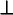c axis) ~=2.59 ( ||c axis) 300 K Goldberg et al.(2001) 6H-SiC ~=2.55 (c axis) ~=2.59 ( ||c axis) 300 K Goldberg et al.(2001) Refractive index n(λ) 3C-SiC n(λ)~= 2.55378 + 3.417 x 104·λ-2 300K, 467nm < λ< 691nm Shaffer & Naum (1969) 4H-SiC n0(λ)~= 2.5610 + 3.4 x 104·λ-2   ne(λ)~= 2.6041 + 3.75 x 104·λ-2 300K, 467nm < λ< 691nm Shaffer & Naum (1971) 6H-SiC n0(λ)~= 2.55531 + 3.34 x 104·λ-2  ne(λ)~= 2.5852 + 3.68 x 104·λ-2 300K, 467nm < λ< 691nm Shaffer & Naum (1971) also see Refractive index n vs. wavelength and photon energy Shaffer & Naum (1971) Radiative recombination coefficient 4H-SiC 1.5 x 10-12 cm3 s-1 300 K, estimate Goldberg et al.(2001) Optical photon energy 3C-SiC 102.8 meV 300 K Goldberg et al.(2001) 4H-SiC 104.2 meV 6H-SiC 104.2 meV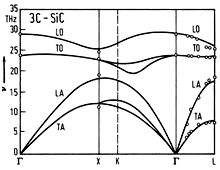3C-SiC. Phonon dispersion relations Derived from an eight-parameter bond-bending force model [Kushawa (1982)]. Circles experimental. [Kushawa (1982)].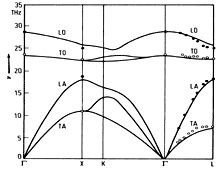3C-SiC. Phonon dispersion relations Derived from a real-space formalism based on scattering theory. Circles experimental. Lee & Joannopoulos (1982)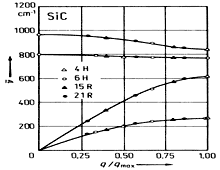4H-SiC, 6H-SiC, 15R-SiC, 21R-SiC. Phonon dispersion relationsvs. reduced wavevector. Along the axial direction Feldman et al. (1982)
 phonon wavenumbers: Remarks Referens 3C-SiC νTO(Γ) 796.2(3) cm-1 T=300K, Raman spectroscopy Olego et al. (1982a) 783-796 cm-1 T=300K Karch et al. (1994), Nakashima & Tahara (1989), Feidman et al. (1968), Olego & Cardona (1982) νLO(Γ) 972.2(3) cm-1 T=300K, Raman spectroscopy Olego et al. (1982a) 829 cm-1 T=300K Karch et al. (1994), Nakashima & Tahara (1989), Feidman et al. (1968), Olego & Cardona (1982) νTA(L) 266 cm-1 T=300K Olego et al. (1982b), Karch et al. (1994), Nakashima & Tahara (1989), Feidman et al. (1968), Olego & Cardona (1982) 261-266 cm-1 νLA(L) 610 cm-1 νTO(L) 765-766 cm-1 νLO(L) 837-838 cm-1 νTA(X) 366-373 cm-1 νLA(X) 629-640 cm-1 νTO(X) 755-761 cm-1 νLO(X) 829 cm-1
 main phonon energies: Remarks Referens 4H-SiC TA1 46.7 meV Freitas (1995) TA2 51.4 meV 53.4 meV LA 76.9 meV 78.8 meV TO1 95.0 meV LO 104.0 meV 104.3 meV 6H-SiC TA 36.3 meV discussion of free exciton replica in wavelength modulated absorption Humphreys (1981) TA1 46.3 meV Humphreys (1981) , Freitas (1995) TA2 53.5 meV LA 53.3 meV Humphreys et al. (1981) 77.0 meV Humphreys et al. (1981), Freitas (1995) TO1 94.7 meV Freitas (1995) TO2 95.6 meV Humphreys et al. (1981), Freitas (1995) LO 104.2 meV Humphreys et al. (1981), Freitas (1995) 104.7 meV Humphreys et al. (1981)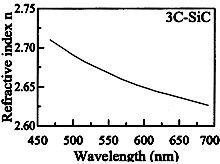3C-SiC. Refractive index n vs. wavelength. 300 K               n(λ)~= 2.55378 + 3.417 x 104·λ-2   Shaffer et al. (1971)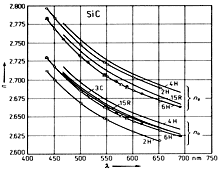2H-SiC, 4H-SiC, 6H-SiC, 15R-SiC. Refractive index vs. wavelength. Powell (1972)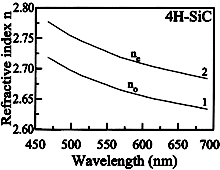4H-SiC. Refractive index n vs. wavelength. 300 K 1 - directionsc axis (n0(λ)); 2 - directions ||c axis (ne(λ)).              n0(λ)~= 2.5610 + 3.4 x 104·λ-2                ne(λ)~= 2.6041 + 3.75 x 104·λ-2 Shaffer et al. (1971)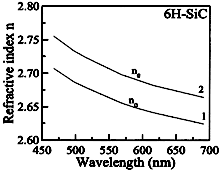6H-SiC. Refractive index n vs. wavelength. 300 K 1 - directionsc axis (n0(λ)); 2 - directions ||c axis (ne(λ)).              n0(λ)~= 2.55531 + 3.34 x 104·λ-2              ne(λ)~= 2.5852 + 3.68 x 104·λ-2 Shaffer et al. (1971)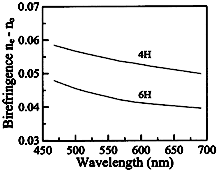4H-SiC, 6H-SiC. Birefringence (ne - n0) vs. wavelength. 300 K Shaffer et al. (1971)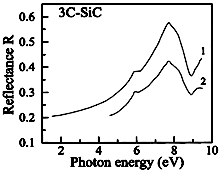3C-SiC. Reflectance R vs. photon energy. 300 K 1 - Logothetidis and Petalas (1996); 2 - Lambrecht et al. (1994)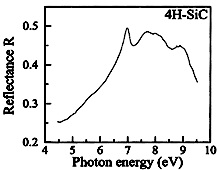4H-SiC. Reflectance R vs. photon energy. 300 K Lambrecht et al. (1993)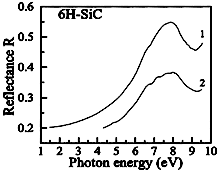6H-SiC. Reflectance R vs. photon energy. 300 K 1 - Logothetidis and Petalas (1996); 2 - Lambrecht et al. (1994)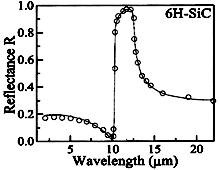6H-SiC. Reflectance R vs. wavelength. 300 Kc axis Spitzer et al. (1959)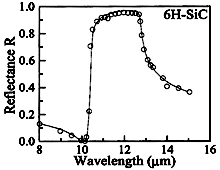6H-SiC. Reflectance R vs. wavelength. 300 K   || c axis Spitzer et al. (1959)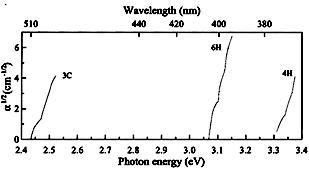3C-SiC, 4H-SiC, 6H-SiC. The absorption coefficient α1/2 vs. photon energy. T=4.2 K T=4.2 K Light-polarized   Ec axis Choyke (1969)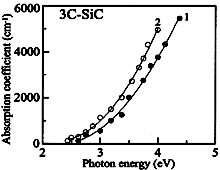3C-SiC. The absorption coefficient vs. photon energy for different electron concentrations T=300 K 1 - Nd = 5 x 1016 cm-3 ; 2 - Nd = 7 x 1016 cm-3 . Solid lines: α = (hν)2 ; Experimental points - Solangi & Chaudhry (1992)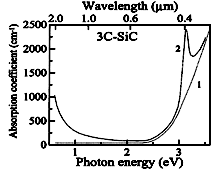3C-SiC. The absorption coefficient vs. photon energy for different electron concentrations T=300 K 1 - relatively pure crystal; 2 - Nd = 1019 cm-3 Patrick & Choyke (1969)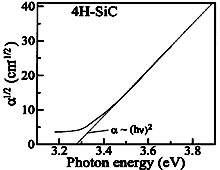4H-SiC. The absorption coefficient vs. photon energy for different electron concentrations T=300 K Low-doped samples.  Ec axis. Sridhara et al. (1998)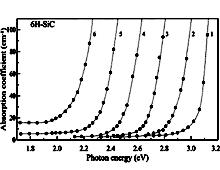6H-SiC. The absorption coefficient vs. photon energy at different temperatures. 1 - T = 293 K (20°C); 2 - T = 573 K (300°C); 3 - T = 873 K (600°C); 4 - T = 1173 K (900°C); 5 - T = 1473 K (1200°C); 6 - T = 1773 K (1500°C). Groth & Kauer (1961)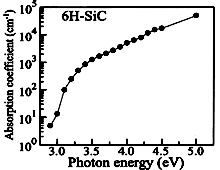6H-SiC. The absorption coefficient vs. photon energy. T = 300 K Philipp & Taft (1960)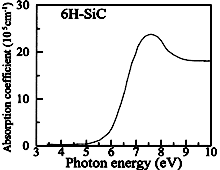6H-SiC. The absorption coefficient vs. photon energy. T = 300 K Philipp & Taft (1960)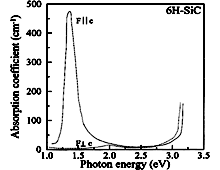6H-SiC. The absorption coefficient vs. photon energy. T = 300 Kc axis and || c axis. Nd - Na ~= 3.4 x 1018 cm-3 Radovanova (1973)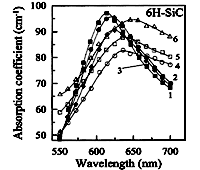6H-SiC. The absorption coefficient vs. photon energy at different temperatures   || c axis, Nd - Na ~= 2.0 x 1018 cm-3. 1 - T = 78 K; 2 - T = 300 K; 3 - T = 390 K; 4 - T = 550 K; 5 - T = 810 K (1200°C); Dubrovskii et al. (1973)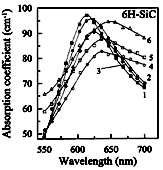6H-SiC. The absorption coefficient vs. photon energy at different temperaturesc axis, Nd - Na ~= 1.0 x 1019 cm-3. 1 - T = 80 K; 2 - T = 300 K; 3 - T = 450 K; 4 - T = 640 K; 5 - T = 930 K; 6 - T = 1100 K; Dubrovskii & Radovanova (1973)6H-SiC doped with B. The absorption coefficient vs. photon energy at different temperatures. 1 - T = 300 K; 2 - T = 400 K; 3 - T = 500 K; 4 - T = 600 K; 5 - T = 700 K; 6 - T = 800 K. Tairov & Tsvetkov (1988)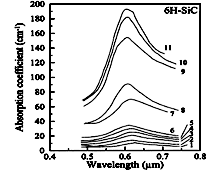6H-SiC. The absorption coefficient vs. wavelength at different Nd - Na values. T = 300 K.c axis 1 - Nd - Na = 1.6 x 1018 cm-3; 2 - Nd - Na = 2.7 x 1018 cm-3; 3 - Nd - Na = 4.0 x 1018 cm-3; 4 - Nd - Na = 4.8 x 1018 cm-3; 5 - Nd - Na = 5.8 x 1018 cm-3; 6 - Nd - Na = 6.3 x 1018 cm-3; 7 - Nd - Na = 1.3 x 1019 cm-3; 8 - Nd - Na = 1.6 x 1019 cm-3; 9 - Nd - Na = 2.6 x 1019 cm-3; 10- Nd - Na = 3.3 x 1019 cm-3; 11- Nd - Na = 4.0 x 1019 cm-3; Radovanova (1973)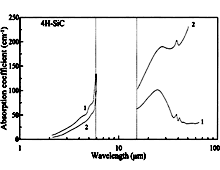4H-SiC. The infrared absorption coefficient vs. wavelength. 1 - T = 80 K; 2 - T = 300 K.   Ec axis.  Nd - Na = 1017 cm-3 Radovanova (1973)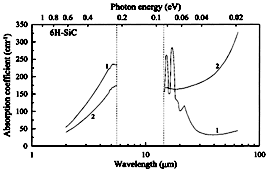4H-SiC. The infrared absorption coefficient vs. wavelength. 1 - T = 80 K; 2 - T = 300 K.   Ec axis.  Nd - Na = 1017 cm-3 Dubrovskii & Radovanova (1971)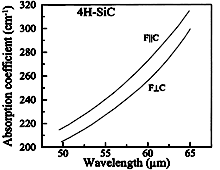4H-SiC. Free carrier absorption coefficient vs. wavelengthc axis and || c axis. T = 300 K.  Nd - Na = 3 x 1017 cm-3 Radovanova (1973)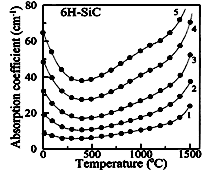6H-SiC. The absorption coefficient vs. temperature at different wavelength λ. 1 - λ = 1.49 µm; 2 - λ = 2.0 µm; 3 - λ = 2.25 µm; 4 - λ = 3.03 µm; 5 - λ = 3.52 µm; Groth & Kauer (1961)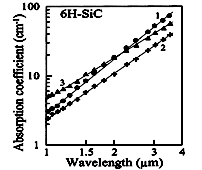6H-SiC. The absorption coefficient α vs. wavelength at different temperature. 1 - α~λ2.6;       - T = 293 K (20°C); 2 - α~λ2.2        - T = 673 K (400°C);    3 - α~λ1.95      - T = 1273 K (1000°C) . Groth & Kauer (1961)